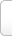﻿ 怕组词_怕字可以怎么组词_能组什么词_有哪些

# 怕 字可以怎么组词

• 怕羞
• 怕不道
• 怕死
• 怕莫
• 怕怕
• 怕事
• 怕死鬼
• 怕痒树
• 怕妇
• 怕不待
• 怕硬欺软
• 怕怖
• 怕怯
• 怕见的是怪，难躲的是债
• 怕人
• 怕痛怕痒
• 怕敢
• 怕不是
• 怕是
• 怕臊
• 怕不
• 怕不大
• 怕生
• 怕不的
• 怕三怕四
• 怕惧
• 怕恐
• 怕死贪生

## “怕”字在中间的能组哪些词

• 人怕老来贫
• 前怕狼，后怕虎
• 好汉只怕病来磨
• 拈轻怕重
• 不怕官，只怕管
• 世上无难事，只怕有心人
• 留得青山在不怕没柴烧
• 初生牛犊不怕虎
• 一年被蛇咬，三年怕草索
• 身正不怕影儿歪
• 欺软怕硬
• 天下无难事，只怕有心人
• 怯红怕黑
• 一年被蛇咬，三年怕井绳
• 树叶掉下来怕打破头
• 人怕出名猪怕壮
• 铁怕落炉，人怕落囤
• 树叶子掉下来都怕打了头
• 不怕不识货，就怕货比货
• 身正不怕影儿斜
• 阎王也怕拚命鬼
• 留得青山在，不怕没柴烧
• 真金不怕火炼
• 真金不怕火
• 白天不做亏心事，半夜不怕鬼敲门
• 鬼怕恶人
• 世上无难事只怕有心人
• 英雄只怕病来磨
• 贪生怕死
• 不怕慢，就怕站
• 酒香不怕巷子深
• 不怕县官，只怕现管
• 一朝被蛇咬，三年怕井绳
• 死猪不怕开水烫
• 一度著蛇咬，怕见断井索
• 身正不怕影子斜
• 欺善怕恶
• 不怕货比货，就怕不识货
• 乳犊不怕虎
• 不怕一万，就怕万一
• 胆小怕事
• 人怕出名猪怕肥
• 好汉不怕出身低
• 贼没种，只怕哄
• 一夜被蛇咬，十日怕麻绳

## “怕”字在结尾组词有哪些

• 心惊胆怕
• 躭惊受怕
• 耽惊受怕
• 吃惊受怕
• 蛇回兽怕
• 慌怕
• 只怕
• 怕怕
• 深怕
• 敢怕
• 後怕
• 惧怕
• 惊怕
• 担惊受怕
• 天不怕，地不怕
• 那怕
• 可怕
• 嫌怕
• 别怕
• 哪怕
• 害怕
• 生怕
• 恐怕
• 鬼见怕
• 担惊忍怕
• 最怕
• 骇怕
• 躭怕
• 吓怕
• 不怕
• 愧怕
• 避怕
• 惔怕

### 怕 的拼音及解释Tip:SCCG